;nbsp

Hi parents and students! This is an introduction to what you’ll be needing to know by the end of the first Algebra 1 semester. My courses cover all of the common core requirements (the syllabus for semester 1 is here, and semester 2 is here!) and prepare my students to think critically. Not only do I want your student to know the material in my class, I want them to succeed in all future classes and opportunities. Please try out this sample test to see what your child will be learning this semester!

Name

This is a required question

Email

Must be a valid email address
This is a required question

1. What are the roots of x*(x-5)*(x+12) = 0
This is a required question

2. Identify the inequality of [ 3 < -x + 4 < 15 ] on a number line.
Solve the inequality first!
This is a required question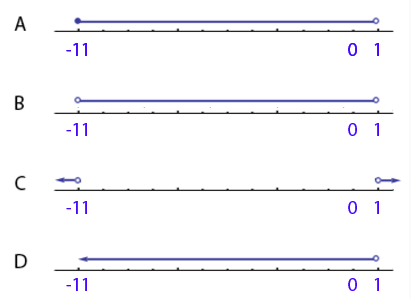3. Identify the graph of the system of inequalities [ x – 2y ≥ 4 ] & [ 3y + x > 18 ]
This is a required question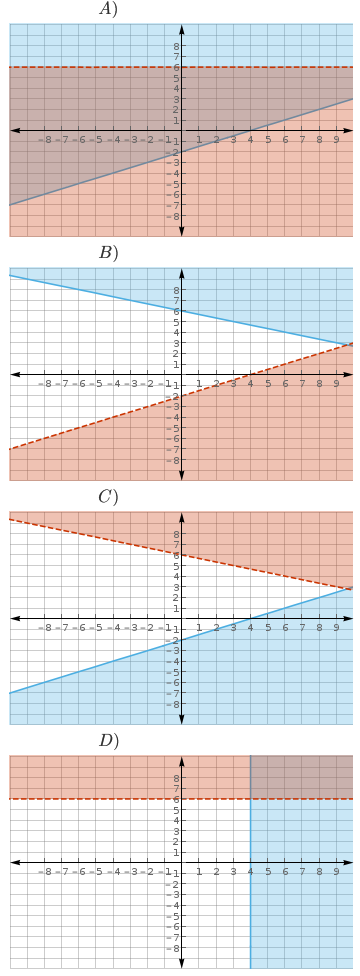4. In a three lap race a driver wishes to have at least a 180 mph average. If his speed on the first lap was 165 mph and 181 mph on the second lap, what must his speed be on the third lap so that the desired average is reached?
Average
This is a required question

5. Factor [ 4x² – 49 ]
This is a required question

6. Expand [ 6x*(x – 8)*(2x + 5) ]
This is a required question

7a. When using the quadratic formula to solve for [ x² = -11x – 30 ], what is the value for b?
This is a required question

7b. Solve [ x² = -11x – 30] using the quadratic formula.
This is a required question

8. Identify the graph of [ y = (5.2)^(x) – 3 ]
This is a required question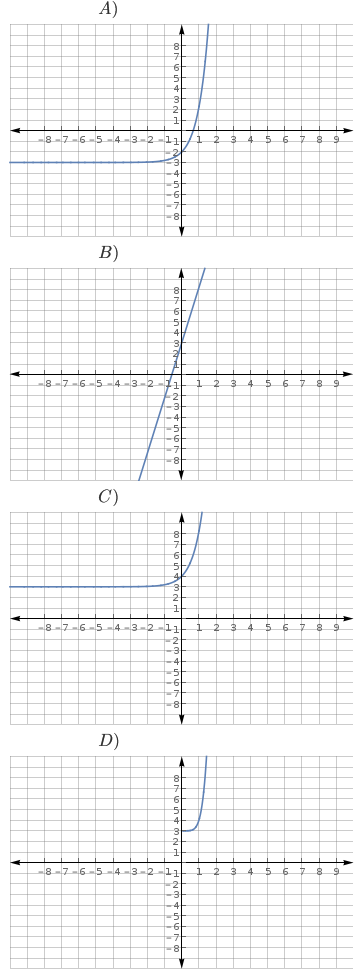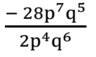9. Simplify [ -28p⁷q⁵/(2p⁴q⁶) ]
This is a required question10. Simplify [ (16a⁴b² – 8a²b² – 2a⁴b²) / 4 ]
Group, factor and cancel
This is a required question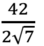11. Simplify [ 42 / (2√7) ]
Remove the root from the denominator
This is a required question

12. Factor [ -11v + 22v² – 121v³ ]
This is a required question

13. Factor [ (-4x²b³)⁴ – (-2x⁴b²)³ ]
Distribute the exponents and find a GCF.
This is a required question

14. Simplify [ (30c⁻⁵) / (12c⁻²) ]
This is a required question

15. Factor [ -2x² – 14x + 36 ]
Box method for factoring
This is a required question

16. Factor [ p² + 2p – 24 ]
Box method for factoring
This is a required question

17. Factor [ w² – 12w + 36 ]
Box method for factoring
This is a required question

18. Factor [ y² + 18y + 81 ]
Box method for factoring
This is a required question

19. Find the zeros of [ 4x² + 35x – 9 = 0 ]
Factor and solve
This is a required question

20. Which of the following equations has a minimum point of (0, -4)?
Think of the graph of a parabola!
This is a required question

21. What are the roots of [ 2x² – 6x + 1 = 0 ]
This is a required question

22. Identify the graphs of [ y = 4x² ] & [ y = -.1x² ] on the same coordinate plane.
This is a required question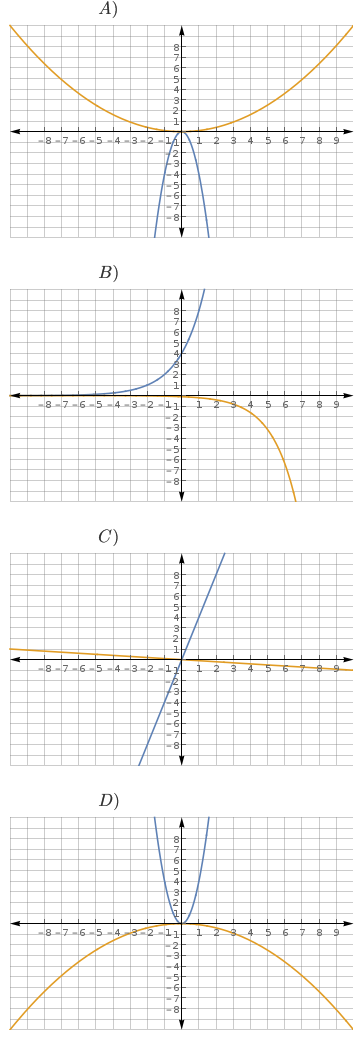23. Simplify [ √24 – 8√54 ]
Can you make them the same root?
This is a required question

24a. Identify the equation of the parabola with stretch factor, a = -2, and a maximum point of (0, -4).
This is a required question

24b. Identify the graph of the equation.
This is a required question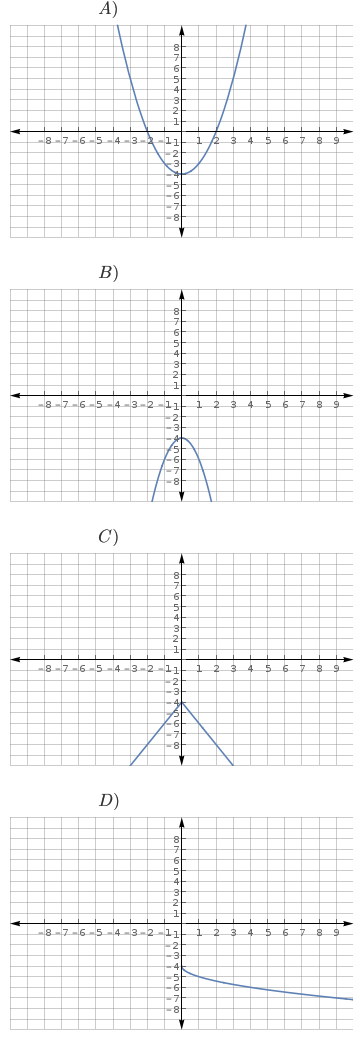25. How many roots does [ y = .5x² – 2x – 7 ] have?
This is a required question

26. A car purchased for \$25,000 will depreciate by 6% per year. How much will it be worth in 4 years?
A(t) = A₀(1 – r)^t
This is a required question

27a. A pile of nickels and quarters is worth \$4.40. There are 32 coins in total. What is the system of equations used to solve this problem?
This is a required question

27b. Solve the system. How many quarters are there?
This is a required question

28. The lawn can be mowed by Bo in 45 minutes while it takes Larry a full hour to do the same lawn. If both work together, how long will it take them to mow the lawn?
Find the rate for each. Add them together and solve.
This is a required question

29. Find the distance between (4.7, -2.8) and (3.02, 17)
d = √((x₁-x₂)² + (y₁-y₂)²)
This is a required question

30. What is the midpoint of the line segment connecting (4, -1) and (2, 15)?
mp = ((x₁ + x₂)/2, (y₁+y₂)/2)
This is a required question

This is a required question